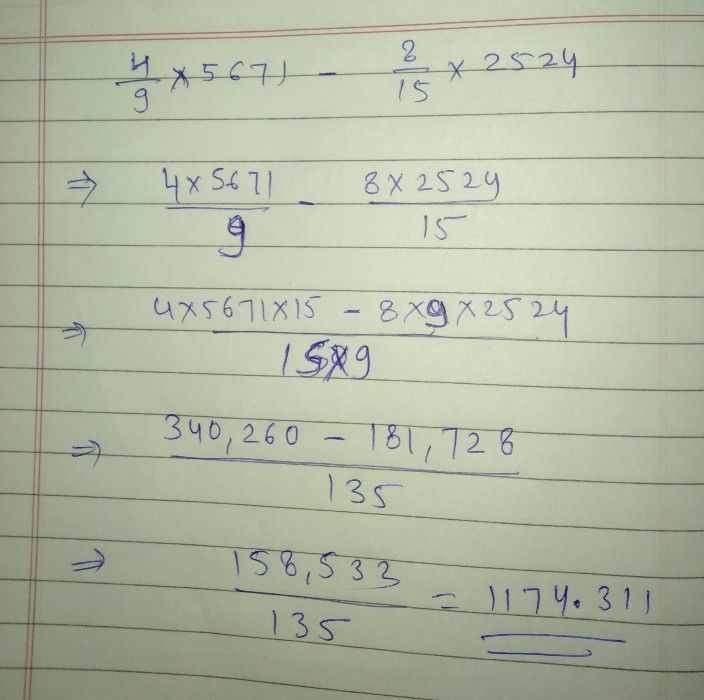Symbol
Problem$10$ $\dfrac {4} {9}\times 5671$ $-\dfrac {8} {15}\times 2524=2$ $△\sqrt{}$
10th-13th grade
Other
SolutionQanda teacher - pawansirStudent
how can I solve this problem without using calculator.....
and ofcourse within 20 to 30 secondsQanda teacher - pawansir
okay
then just dived 5671 by 9 and 2524 by 15 then you'll getStudent
thanks